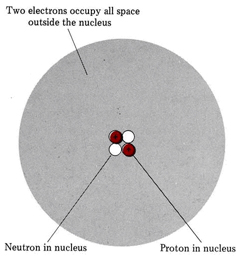# 3.11: Atomic Structure and Isotopes

$$\newcommand{\vecs}{\overset { \rightharpoonup} {\mathbf{#1}} }$$ $$\newcommand{\vecd}{\overset{-\!-\!\rightharpoonup}{\vphantom{a}\smash {#1}}}$$$$\newcommand{\id}{\mathrm{id}}$$ $$\newcommand{\Span}{\mathrm{span}}$$ $$\newcommand{\kernel}{\mathrm{null}\,}$$ $$\newcommand{\range}{\mathrm{range}\,}$$ $$\newcommand{\RealPart}{\mathrm{Re}}$$ $$\newcommand{\ImaginaryPart}{\mathrm{Im}}$$ $$\newcommand{\Argument}{\mathrm{Arg}}$$ $$\newcommand{\norm}{\| #1 \|}$$ $$\newcommand{\inner}{\langle #1, #2 \rangle}$$ $$\newcommand{\Span}{\mathrm{span}}$$ $$\newcommand{\id}{\mathrm{id}}$$ $$\newcommand{\Span}{\mathrm{span}}$$ $$\newcommand{\kernel}{\mathrm{null}\,}$$ $$\newcommand{\range}{\mathrm{range}\,}$$ $$\newcommand{\RealPart}{\mathrm{Re}}$$ $$\newcommand{\ImaginaryPart}{\mathrm{Im}}$$ $$\newcommand{\Argument}{\mathrm{Arg}}$$ $$\newcommand{\norm}{\| #1 \|}$$ $$\newcommand{\inner}{\langle #1, #2 \rangle}$$ $$\newcommand{\Span}{\mathrm{span}}$$$$\newcommand{\AA}{\unicode[.8,0]{x212B}}$$

The experimental facts described in the preceding section can be accounted for by assuming that any atom is made up of three kinds of subatomic particles.

1. The electron carries a charge of –e, has a mass about $$\tfrac{1}{1837}$$ that of a hydrogen atom, and occupies most of the volume of the atom.
2. The proton carries a charge of +e, has a mass about the same as a hydrogen atom, and is found within the very small volume of the nucleus.
3. The neutron carries no electric charge, has about the same mass as a hydrogen atom, and is found in the nucleus.

Some important properties of the three kinds of subatomic particles are listed in the following table. Experimental evidence for the existence of the neutron was first correctly interpreted in 1932 by James Chadwick (1891 to 1974), a discovery for which he was awarded the Nobel Prize in 1935.

Table $$\PageIndex{1}$$: Important Subatomic Particles and Some of Their Properties.
Particle
Mass (kg)
Electric Charge (C)
Location
Electron 9.1095 × 10–31 –1.6022 × 10–19
Outside nucleus
Proton 1.6726 × 10–27 +1.6022 × 10–19
In nucleus
Neutron 1.6750 × 10–27
0
In nucleus

The modern picture of a helium atom, which is made up of two electrons, two protons, and two neutrons, is shown below. Because each proton and each neutron has more than 1800 times the mass of an electron, nearly all the mass of the helium atom is accounted for by the nucleus. This agrees with Rutherford’s interpretation of the Geiger-Marsden experiment.Figure $$\PageIndex{1}$$: The atomic structure of a helium atom. Two electrons, two protons, and two neutrons are arranged as shown.

The number of units of positive charge on the nucleus is usually about half the number of units of mass because about half the nuclear particles are uncharged neutrons. The two electrons move about rapidly, occupying all the volume of the atom outside the nucleus. Their negative charge neutralizes the positive charge of the two protons, producing a neutral or uncharged atom.

The protons and neutrons in the nucleus of an atom such as helium are held very tightly by strong nuclear forces. It is very difficult either to separate the nuclear particles or to add extra ones. The electrons, on the other hand, are held to the atom by their electrostatic attraction for the positively charged protons in the nucleus. This force is strong, but not so strong that an atom cannot lose or gain electrons. When the number of electrons is not the same as the number of protons, an atom has a net electric charge and is called an ion. The $$α$$ particles emitted by radioactive elements consist of two protons and two neutrons tightly bound together. Thus an $$α$$ particle is the same as a helium nucleus; that is, a helium atom that has lost its two electrons or a helium ion whose charge is +2e. When particles are emitted into a closed container, they slowly pick up electrons from their surroundings, and eventually the container becomes filled with helium.

The structure of any atom may be specified by indicating how many electrons, protons, and neutrons it contains. The number of protons is the same as the number of electrons and is given by the atomic number Z. Instead of directly specifying how many neutrons are present, we use the mass number $$A$$. This is the total number of particles in the nucleus; hence

\begin{align*}A &= \text{number of protons} + \text{number of neutrons}\\[4pt] &= Z + N \end{align*} \nonumber

where $$N$$ represents the number of neutrons. To symbolize a particular atom, the mass number and atomic number are written as a superscript and subscript preceding the chemical symbol ($$\ce{Sy}$$) as follows:

${}_{Z}^{A}\text{Sy} \nonumber$

The helium atom, whose structure was represented above, has 2 protons and 2 electrons (Z = 2)as well as 2 neutrons. Hence A = Z + N = 2 + 2 = 4, and the atom is represented by

${}_{2}^{4}\text{He} \nonumber$

In the case of an ion the positive or negative charge is indicated as a superscript to the right of the chemical symbol. Thus a helium atom which had lost two electrons (a helium ion with two more protons than electrons) would be written as

${}_{2}^{4}\text{He}^{\text{2+}} \nonumber$

##### Example $$\PageIndex{1}$$ : Atomic Numbers

How many electrons, protons, and neutrons are there in each of the atoms represented below?

1. $${}_{\text{6}}^{\text{12}}\text{C}$$
2. $${}_{\text{20}}^{\text{40}}\text{Ca}$$
3. $${}_{\text{82}}^{\text{206}}\text{Pb}$$
4. $${}_{\text{20}}^{\text{40}}\text{Ca}^{\text{2+}}$$
###### Solution

For an atom the number of electrons equals the number of protons and is given by Z. For an ion the atomic number gives the number of protons, but the number of electrons must be determined from the charge. Thus

1. $${}_{\text{6}}^{\text{12}}\text{C}$$ contains 6 electrons and 6 protons.
2. $${}_{\text{20}}^{\text{40}}\text{Ca}$$ contains 20 electrons and 20 protons.
3. $${}_{\text{82}}^{\text{206}}\text{Pb}$$ contains 82 electrons and 82 protons.
4. $${}_{\text{20}}^{\text{40}}\text{Ca}^{\text{2+}}$$ has lost two electrons. Therefore it contains 18 electrons and 20 protons.

The number of neutrons can be obtained by subtracting the number of protons (Z) from the total number of particles in the nucleus (A):

1. $${}_{\text{6}}^{\text{12}}\text{C}$$: N = A – Z = 12 – 6 = 6 neutrons
2. $${}_{\text{20}}^{\text{40}}\text{Ca}$$: N = 40 – 20 = 20 neutrons
3. (The same applies to $${}_{\text{20}}^{\text{40}}\text{Ca}^{\text{2+}}$$. Only electrons are gained or lost when an ion forms.)
4. $${}_{\text{82}}^{\text{206}}\text{Pb}$$: N = 206 – 82 = 124 neutrons

This page titled 3.11: Atomic Structure and Isotopes is shared under a CC BY-NC-SA license and was authored, remixed, and/or curated by Ed Vitz, John W. Moore, Justin Shorb, Xavier Prat-Resina, Tim Wendorff, & Adam Hahn.